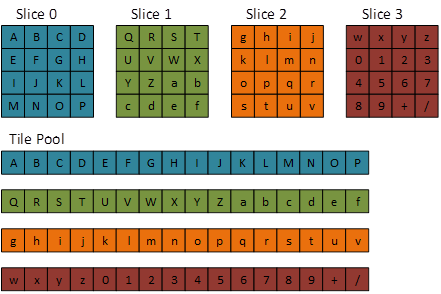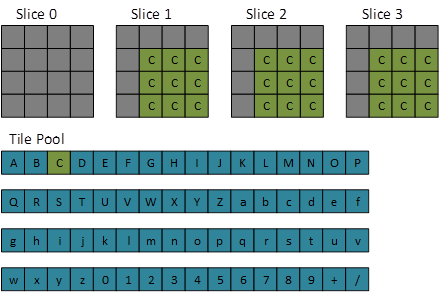# Volume tiled resources (Direct3D 12)

Volume (3D) textures can be used as tiled resources, noting that tile resolution is three-dimensional.

## Overview

Tiled resources decouple a Direct3D resource object from its backing memory (resources in the past had a 1:1 relationship with their backing memory). This allows for a variety of interesting scenarios such as streaming in texture data and reusing or reducing memory usage.

2D texture tiled resources are supported in Direct3D 11.2. Optional support for 3D tiled textures is available for Direct3D 12 and Direct3D 11.3 (refer to D3D12_TILED_RESOURCES_TIER).

The typical resource dimensions used in tiling are 4 x 4 tiles for 2D textures, and 4 x 4 x 4 tiles for 3D textures.

Bits/pixel (1 sample/pixel) Tile dimensions (pixels, w x h x d)
8 64x32x32
16 32x32x32
32 32x32x16
64 32x16x16
128 16x16x16
BC 1,4 128x64x16
BC 2,3,5,6,7 64x64x16

Note the following formats are not supported with tiled resources: 96bpp formats, video formats, R1_UNORM, R8G8_B8G8_UNORM, R8R8_G8B8_UNORM.

In the diagrams below, dark gray represents NULL tiles.

### Texture 3D tiled resource default mapping (most-detailed mip)### Texture 3D tiled resource default mapping (second-most-detailed mip)### Texture 3D tiled resource (most-detailed mip)

The following code sets up a 3D tiled resource at the most-detailed mip.

``````D3D12_TILED_RESOURCE_COORDINATE trCoord{};
trCoord.X = 1;
trCoord.Y = 0;
trCoord.Z = 0;
trCoord.Subresource = 0;

D3D12_TILE_REGION_SIZE trSize{};
trSize.bUseBox = false;
trSize.NumTiles = 63;
``````### Texture 3D tiled resource (second-most-detailed mip)

The following code sets up a 3D tiled resource, and the second most detailed mip.

``````D3D12_TILED_RESOURCE_COORDINATE trCoord;
trCoord.X = 1;
trCoord.Y = 0;
trCoord.Z = 0;
trCoord.Subresource = 1;

D3D12_TILE_REGION_SIZE trSize;
trSize.bUseBox = false;
trSize.NumTiles = 6;
``````### Texture 3D tiled resource (single tile)

The following code sets up a single tile resource.

``````D3D12_TILED_RESOURCE_COORDINATE trCoord;
trCoord.X = 1;
trCoord.Y = 1;
trCoord.Z = 1;
trCoord.Subresource = 0;

D3D12_TILE_REGION_SIZE trSize;
trSize.bUseBox = true;
trSize.NumTiles = 27;
trSize.Width = 3;
trSize.Height = 3;
trSize.Depth = 3;
``````### Texture 3D tiled resource (uniform box)

The following code sets up a uniform box tiled resource (note the statement `trSize.bUseBox = true;) :`

``````D3D12_TILED_RESOURCE_COORDINATE trCoord;
trCoord.X = 0;
trCoord.Y = 1;
trCoord.Z = 0;
trCoord.Subresource = 0;

D3D12_TILE_REGION_SIZE trSize;
trSize.bUseBox = true;
trSize.NumTiles = 27;
trSize.Width = 3;
trSize.Height = 3;
trSize.Depth = 3;
``````## Tiled resource APIs

The same API calls are used for both 2D and 3D tiled resources.

Enums

Structs

Methods